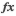# MemoryBuffer

## A buffer of memory. Can be used in IO or Import calls. You can write to this or read from it, which moves the cursor within the buffer.

A buffer of memory. Can be used in IO or Import calls. You can write to this or read from it, which moves the cursor within the buffer.

Behaviors`MemoryBuffer(const MemoryBuffer&in)``MemoryBuffer()``MemoryBuffer(uint64 size)``MemoryBuffer(uint64 size, uint8 init)``~MemoryBuffer()`

Methods`bool AtEnd()``uint64 GetSize()``double ReadDouble()``float ReadFloat()``int16 ReadInt16()``int ReadInt32()``int64 ReadInt64()``int8 ReadInt8()``string ReadString(uint64 size)``uint16 ReadUInt16()``uint ReadUInt32()``uint64 ReadUInt64()``uint8 ReadUInt8()``void Resize(uint64 size)``void Seek(uint64 pos, int dir = 0)``void Write(const string&in str)``void Write(const float&in i)``void Write(const int64&in i)``void Write(const int&in i)``void Write(const int16&in i)``void Write(const int8&in i)``void Write(const uint64&in i)``void Write(const uint&in i)``void Write(const uint16&in i)``void Write(const uint8&in i)``void Write(const double&in i)`